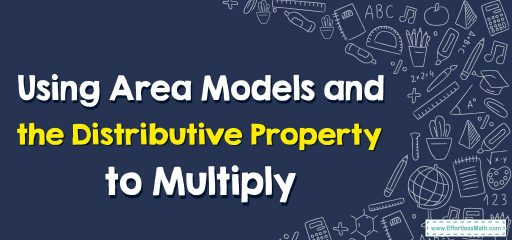# How to Use Area Models and the Distributive Property to Multiply?

Multiplying using area models and the distributive property is a visual and conceptual way to understand multiplication.## A Step-by-step Guide to Use Area Models and the Distributive Property to Multiply

Here are the steps to use these methods:

### Step 1: Understand the Area Model:

An area model is a rectangle that represents the two numbers being multiplied.

The length of the rectangle represents one factor, and the width represents the other. The area of the rectangle represents the product.

### Step 2: Break down the Numbers:

To use the distributive property, you need to break down the numbers into smaller parts.

### Step 3: Use the Distributive Property:

Once you have broken down the numbers, you can use the distributive property to multiply.

### Step 4: Draw the Area Model:

To use the area model, draw a rectangle with the length and width representing the two factors. Then divide the rectangle into smaller rectangles to match the parts from the distributive property.

### Step 5: Multiply and Add:

Finally, multiply each part separately and then add the products together to get the final product.

### Using Area Models and the Distributive Property to Multiply – Example 1:

Use the area model to multiply $$24 × 4$$.

#### Solution:

Step 1: Break down the numbers: $$24 = 20 + 4$$

Step 2: Use the distributive property: $$24 × 4 = (20 × 4) + (4 × 4)$$

Step 3: Draw the area model with smaller rectangles:

$$20 × 4 = 80$$

$$4 × 4 = 16$$

$$80 + 16 = 96$$

Therefore, $$24 × 4 = 96$$.

### What people say about "How to Use Area Models and the Distributive Property to Multiply? - Effortless Math: We Help Students Learn to LOVE Mathematics"?

No one replied yet.

X
30% OFF

Limited time only!

Save Over 30%

SAVE $5 It was$16.99 now it is \$11.99